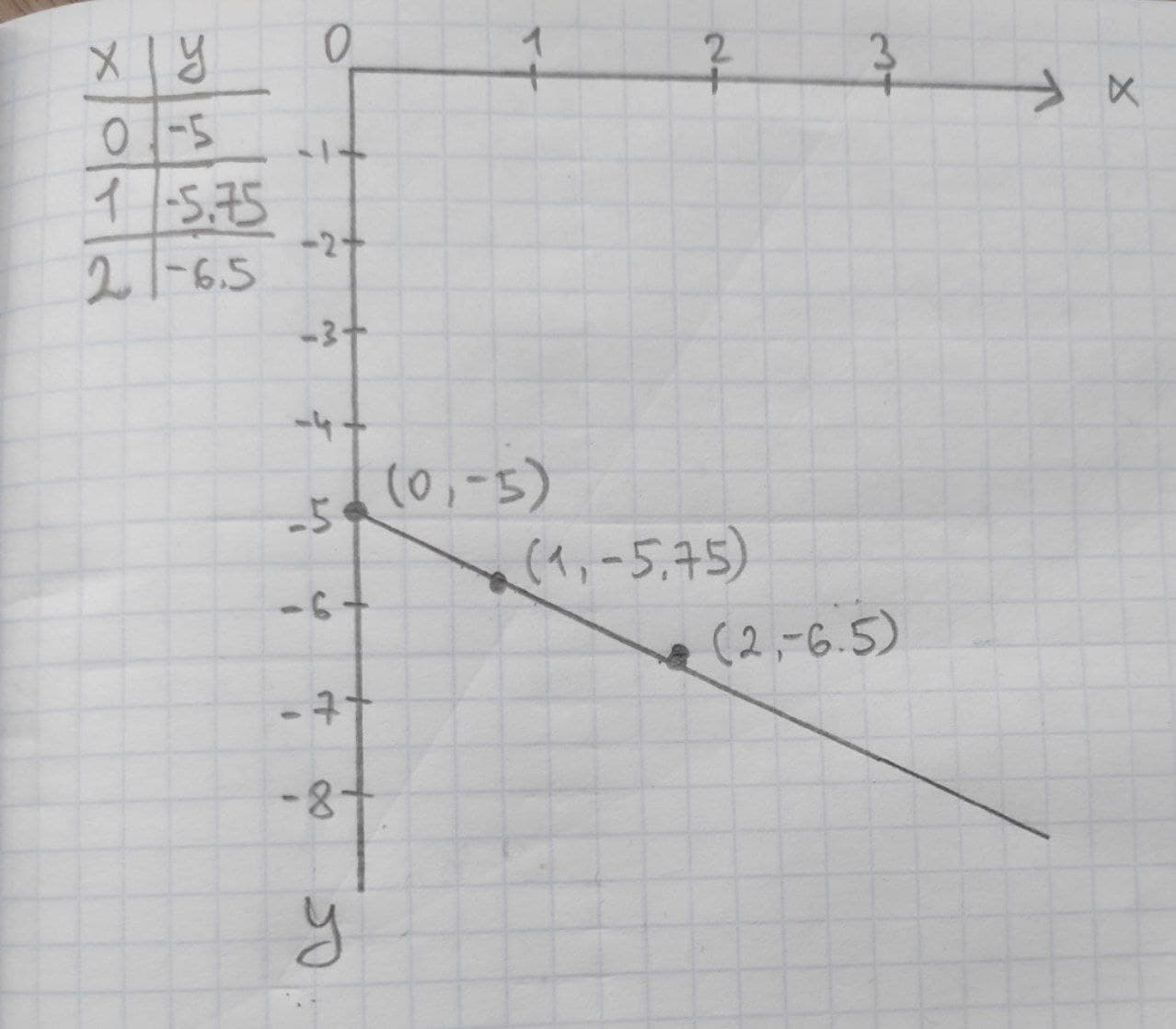Given linear equation y = -0.75x-5 a. find the y-intercept and slope. b. determine whether the line slopes upward, slopes downward, or is horizontal, without graphing the equation. c. use two points to graph the equation.Braxton Pugh 2020-11-05 Answered
Given linear equation y = -0.75x-5
a. find the y-intercept and slope.
b. determine whether the line slopes upward, slopes downward, or is horizontal, without graphing the equation.
c. use two points to graph the equation.

• Questions are typically answered in as fast as 30 minutes

Solve your problem for the price of one coffee

• Math expert for every subject
• Pay only if we can solve itunett
(a) $$\displaystyle{y}={b}_{{0}}+{b}_{{1}}{x}$$
where $$\displaystyle{b}_{{0}}$$ is the y intercept
$$\displaystyle{b}_{{1}}$$ is the slope
Slope = -0.75
y-intercept = -5
from the linear equation
$$\displaystyle{b}_{{0}}=-{5}$$
$$\displaystyle{b}_{{1}}=-{0.75}$$
(b) The slope is downward in graph. Because, the slope is negative value.
(c) Two point to graph: